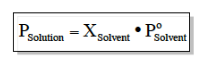# Problem: A solution is made by dissolving 25.8 g urea (CH4N2O), a nonelectrolyte, in 275 g water. Calculate the vapor pressures of this solution at 25°C and 45°C. (The vapor pressure of pure water is 23.8 torr at 25°C and 71.9 torr at 45°C.)

###### FREE Expert Solution

We can calculate the vapor pressure of the solution using the equation below:X is the mole fraction of the solute. We don't have that value readily available yet so we have to calculate it through multiple steps. The solute is the one in greater amounts. In this problem it's water.

84% (156 ratings)###### Problem Details

A solution is made by dissolving 25.8 g urea (CH4N2O), a nonelectrolyte, in 275 g water. Calculate the vapor pressures of this solution at 25°C and 45°C. (The vapor pressure of pure water is 23.8 torr at 25°C and 71.9 torr at 45°C.)